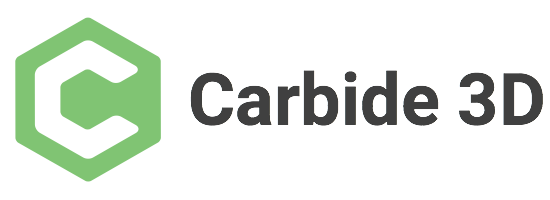# Is it possible to draw a semi circle in CC?

I only want a little over 3/4 of a circle. Can I draw a circle, then overlap a square, boolean to get the right amount of circle then “break” the object as you would in autocad?

Sure. I would draw the circle then draw a square with sides greater than the radius of the circle; move the square so one corner is at the center of the circle; duplicate the square and rotate to get the desired angle (either union or intersect the two squares to get the desired angle); then remove the shape created from the two squares from the circle.